# How many unique 1H NMR signals exist in the spectrum of the following compound?

How many unique 1H NMR signals exist in the spectrum of the following compound?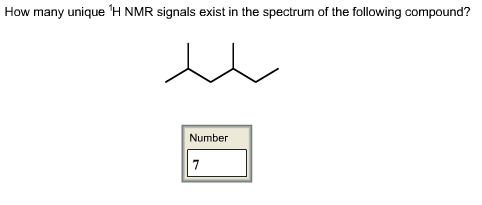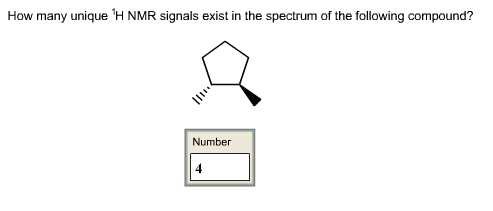Concepts and reason
1H NMR Spectroscopy:

• Nuclear magnetic resonance (NMR)spectroscopy is one of the techniques in analytical chemistry that is widely used to determine the purity of a sample and also to predict the structure of the organic compounds.
• The NMR spectroscopy is a phenomenon that is observed, when frequency of nuclei of atoms (sample) resonates with frequency of the rotating magnetic field.
• The 1H NMR spectroscopy provides the information about how many types of hydrogens (chemically non-equivalent hydrogens) are present in the atom of a molecule.
• 1H NMR gives the information about chemically non-equivalent protons present in the molecules.
• The resonance frequency is observed with reference to the standard compound (0 ppm) which is represented as chemical shift.
• When the protons have chemically same environment, same chemical shift value is observed and the chemical shift value is measured in ppm.

Fundamentals
The protons have chemically same environment.
For example: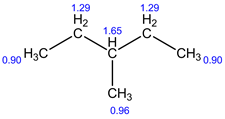In the above structure, all the six carbons have proton(s) but only 4 signals are observed in 1H NMR because the right and left side of the protons have chemically same environment with respect to the third carbon (middle one). Therefore, it show only four signals.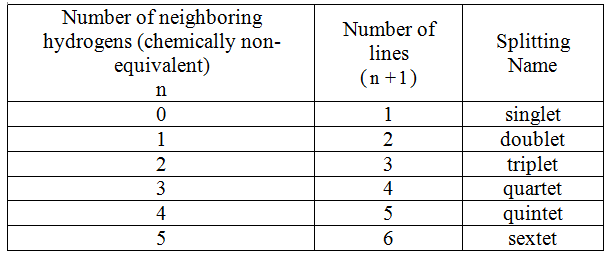1H NMR:13C NMR: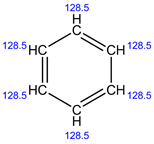Explanation:
In 1H NMR, all the 6 protons have same environment. Therefore, only 1 signal is present.
In 13C NMR, all the 6 carbons have the same environment. Therefore, the same chemical shift value is observed and shows only 1 signal.

1H NMR: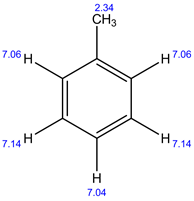13C NMR: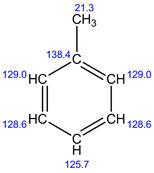Explanation:
In 1H NMR, there are 4 different set of protons present. Therefore, 4 signals are observed.
In 13C NMR, 5 signals are observed. Because, there are 5 sets of carbons present in the given structure.

1H NMR: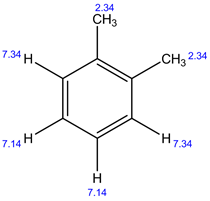13C NMR: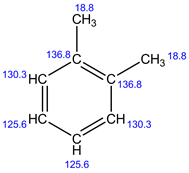Explanation:
In 1H NMR, there are 3 different set of protons present. Therefore, 3 signals are observed.
In 13C NMR, 4 signals are observed. Because, there are 4 set of carbons present in the given structure.

1H NMR: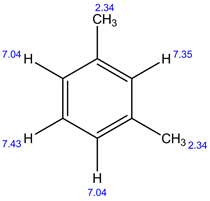13C NMR: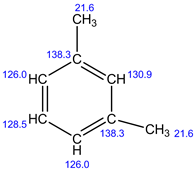Explanation:
In 1H NMR, there are 4 different set of protons present. Therefore, 4 signals are observed.
In 13C NMR, 5 signals are observed. Because, there are 5 sets of carbons present in the given structure.

1H NMR: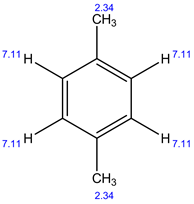13C NMR: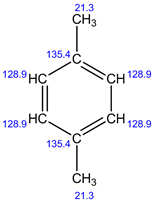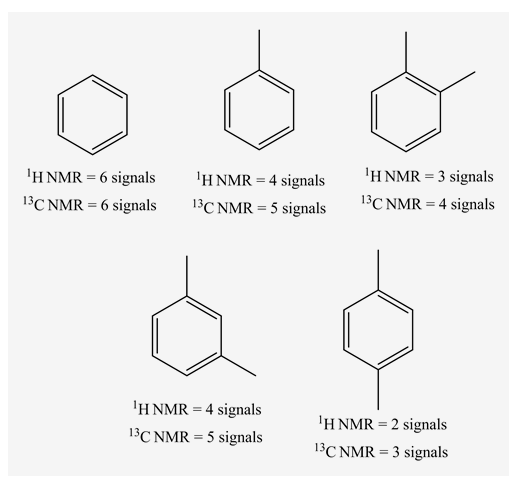Explanation:
In 1H NMR, there are 2 different sets of protons present. Therefore, 2 signals are observed.
In 13C NMR, 3 signals are observed. Because, there are 3 sets of carbons present in the given structure.